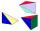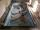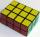# Body volume + surface area - examples - page 3

1. PyramidCuboid ABCDEFGH has dimensions AB 3 cm, BC 4 cm, CG 5 cm. Calculate the volume and surface area of a triangular pyramid ADEC.
2. Surface of the cylinderCalculate the surface area of the cylinder when its volume is 45 l and the perimeter of base is three times of the height.
3. Cone containerRotary cone-shaped container has a volume 1000 cubic cm and a height 12 cm. Calculate how much metal we need for making this package.
4. Pool coatingHow many pieces of tiles 25 cm × 15 cm need to coat the bottom and side walls of the pool with bottom dimensions 30 m × 5 m, if the pool can fit up to 271500 liters of water?
5. Cube wallCalculate the cube's diagonal diagonal if you know that the surface of one wall is equal to 36 centimeters square. Please also calculate its volume.
6. Surface areaThe volume of a cone is 1000 cm3 and the content area of the axis cut is 100 cm2. Calculate the surface area of the cone.
7. Triangular pyramidDetermine the volume and surface area of a regular triangular pyramid having a base edge a=20 cm and a lateral edge b = 35 cm
8. Tin with oilTin with oil has the shape of a rotating cylinder whose height is equal to the diameter of its base. Canned surface is 1884 cm2. Calculate how many liters of oil is in the tin.
9. Cylinder surface, volumeThe area of the cylinder surface and the cylinder jacket are in the ratio 3: 5. The height of the cylinder is 5 cm shorter than the radius of the base. Calculate surface area and volume of cylinder.
10. The cylindrical containerThe container has a cylindrical shape the base diameter 0.8 meters has a content area of the base is equal to the content area of the shell. How many full liters of water can be poured maximally into the container?
11. Tetrahedral prism - rhomboid baseCalculate the area and volume tetrahedral prism that has base rhomboid shape and its dimensions are: a = 12 cm, b = 70 mm, v_a = 6 cm, v_h = 1 dm.
12. Cuboid - simpleCalculate the surface area and volume of a cuboid if a = 8 cm, b = 14 cm and c = 6 cm.
13. Hexagonal pyramidRegular hexagonal pyramid has dimensions: length edge of the base a = 1.8 dm and the height of the pyramid = 2.4 dm. Calculate the surface area and volume of a pyramid.
14. Pyramid in cubeIn a cube with edge 12 dm long we have inscribed pyramid with the apex at the center of the upper wall of the cube. Calculate the volume and surface area of the pyramid.
15. Cuboid - volume and areasThe cuboid has a volume of 250 cm3, a surface of 250 cm2 and one side 5 cm long. How do I calculate the remaining sides?
16. Water in aquariumThe aquarium cuboid shape with a length of 25 cm and a width of 30 cm is 9 liters of water. Calculate the areas which are wetted with water.
17. FlowerbedThe flowerbed has a length 3500mm and a width 1400mm. How many foil is needed to covers the flowerbed? How many m2 of foil was consumed for its production (add 10% of the material to the joint and waste)? How many liters of air is inside the enclosure? (F
18. Cuboid wallsCalculate the volume of the cuboid if its different walls have area of 195cm², 135cm² and 117cm².
19. Hexa prismDetermine the volume of hex prism with edge base 4 cm. The body height is 28 cm.
20. Surface and volume od cuboidContent area of the square base of cuboid is Sp = 36 cm2 and its height 80 mm. Determine its surface area and volume.

Do you have an interesting mathematical example that you can't solve it? Enter it, and we can try to solve it.

To this e-mail address, we will reply solution; solved examples are also published here. Please enter e-mail correctly and check whether you don't have a full mailbox.